# Coulomb's Law Formula

Coulomb's Law Formula

Objects with electric charge attract and repel each other by exerting forces. Charges with the same sign repel, and charges with opposite signs attract. The magnitude of the electrostatic force between charges can be found using Coulomb's Law. The electrostatic force depends on the magnitude of the charges, the distance between them, and the Coulomb constant, which is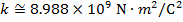. The Coulomb constant can also be written in terms of the permittivity of free space,. In that form, the Coulomb constant is. The values of the electric charges have units of Coulombs, C. Charges are often written as multiples of the smallest possible charge,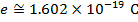. The unit of the electrostatic force is Newtons (N).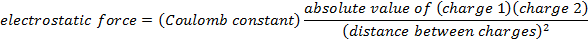F = electrostatic force between two point charges ()

k = Coulomb constant ()

q1 = charge of the first point charge (C)

q2 = charge of the second point charge (C)

r = distance between charges (m)

Coulomb's Law Formula Questions:

1) Two small charged spheres are placed 0.300 m apart. The first has a charge of -3.00 µC (micro-Coulombs), and the second has a charge of -12.0 µC. Do these charged spheres attract or repel? What is the magnitude of the electrostatic force on each sphere?

Answer: The spheres have charges with the same sign, and so the force between them is repulsive. The direction of the force on each sphere points away from the other. To find the magnitude of the force, the charge on the particles must be converted to Coulombs. The prefix "µ", meaning "micro", indicates that the number is scaled by 10-6, and so 1 µC = 10-6 C. The charge of the first sphere is:

q1 = -3.00 µC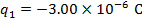The charge of the second sphere is: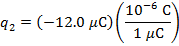The magnitude of the electrostatic force on each sphere can be found using Coulomb's Law: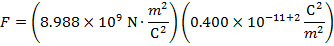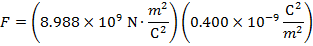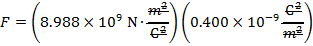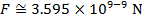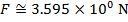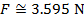The magnitude of the force on each sphere is 3.595 N (Newtons).

2) An electron and a proton are 1.000 nm (nanometer) from each other. The charge of an electron is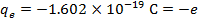, and the charge of a proton is. Do these charges attract or repel? What is the magnitude of the electrostatic force on these charged particles?

Answer: The electron and proton have charges with opposite signs, and so the force between them is attractive. The direction of the force on each particle is in the direction of the other. To find the magnitude of the force, the distance between the particles must first be converted to meters. The prefix "n", meaning "nano", indicates that the number is scaled by 10-9, and so 1 nm = 10-9 m.The distance between the charged particles is:

r = 1.000 nmThe magnitude of the electrostatic force between the particles can be found using Coulomb's Law: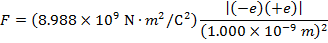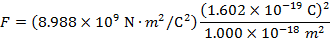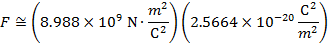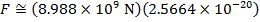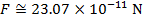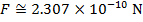The magnitude of the force between the electron and proton is 2.307 x 10-10 N (Newtons).